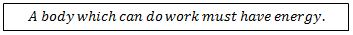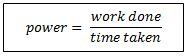# Work, Energy & Power

## Introduction

Much scientific invention has been directed towards designing machines that are beneficial to everyday life. For example, the invention of automobiles completely transformed society. In order for automobiles to work for us, they need to be powerful and energy efficient. Work, energy, power and efficiency are important terms in analysing the performance of machines.

A. Work

Work is done when a force moves to the point at which it acts (the point of application) in the direction of the force. When a force moves its point of application in the direction of the force, the force does work and the work done by the force is said to be positive. Conversely, if the direction of the force is opposite to the direction of movement, work is done on the force. This work done is then said to be negative.An alternative name for distance moved in a particular direction is ‘displacement’. Displacement is a vector quantity, as is force. However, work done has no direction, only magnitude (size), and is a scalar quantity. It is measured in joules (J).Figure 1 illustrates the above principle that one joule of work is done when 1 N force lifts an object by 1 m.Figure 1: Work Done

B. Energy

In order to wind up a spring, work has to be done because a force must be moved through a distance. When the spring is wound, it stores the ability to do work. Anything that is able to do work is said to have energy. Energy is a scalar quantity. It is measured in joules (J).C. Power

Machines such as wind turbines or engines do work when they change energy into a useful form. The rate of converting energy or using energy is known as power. Consider an automobile engine. It converts chemical energy stored in the form of fuel into mechanical energy in the form rotational movement. Power is given by the formula:The unit of power is called watt (W). One watt is equal to a rate of working of 1 joule per second. Power is a scalar quantity. Consider a force (F), which moves a distance (x) at constant velocity (v) in the direction of the force, in time (t). The work done (W) by the force is given by the following equation:Another important term in power is ‘kilowatt-hour’. One kilowatt-hour is the energy expended when work is done at the rate of 1 kilowatt for a time of 1 hour.

D. Efficiency

Efficiency is a measure of how much of a total energy spend may be considered useful and is not lost. Efficiency may be given as a ratio or as a percentage. Since energy cannot be created, efficiency can never be greater than 100%.Consider a hairdryer that consumes 1000 J of electrical energy, but it only produces 350 J of heat, that is a useful form of energy, from that machine. As per the above formula, the efficiency of the hairdryer is 35%. Machines with 100% efficiency are called perpetual machines. In reality, perpetual machines are impossible to design.Figure 2: Efficiency

E. Summary

• Work is done when a force moves something to the point at which it acts (the point of application) in the direction of the force.
• Work done = force x distance moved by the force in the direction of the force.
• Energy is needed to do work; energy is the ability to do work.
• The rate of converting energy or using energy is known as power.
• 1 W is equal to a rate of working of 1 joule per second.
• 1 kilowatt-hour is the energy expended when work is done at the rate of 1 kilowatt for a time of 1 hour.
• Efficiency is a measure of how much of a total energy spend may be considered useful and is not lost.